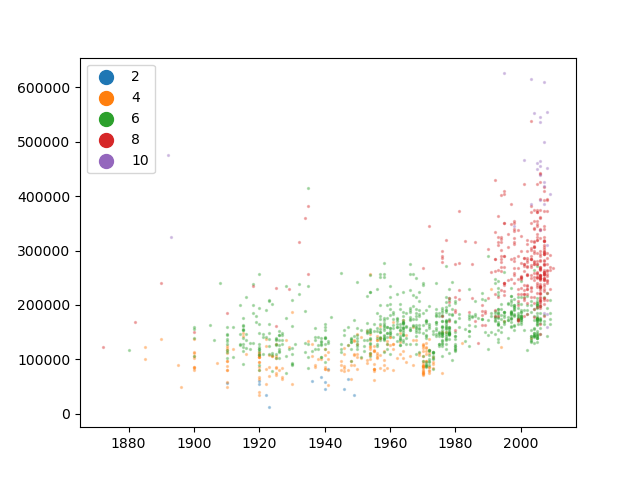# Discrete Scatter Example¶```import matplotlib.pyplot as plt
from dabl.plot import discrete_scatter

# Scatter plot for year built and house price grouped by category of quality.
discrete_scatter(
x=data["Year Built"],
y=data["SalePrice"],
c=data["Overall Qual"],
unique_c=[2, 4, 6, 8, 10],
legend=True,
alpha=0.3
)
plt.show()
```

Total running time of the script: ( 0 minutes 0.181 seconds)

Gallery generated by Sphinx-Gallery